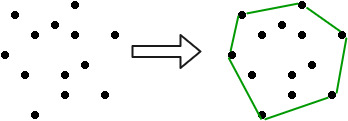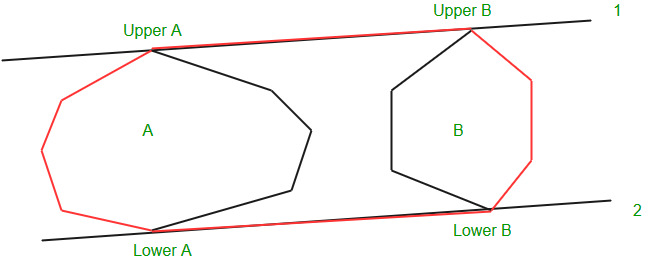# Convex Hull using Divide and Conquer Algorithm

• Difficulty Level : Hard
• Last Updated : 13 Sep, 2018

A convex hull is the smallest convex polygon containing all the given points.Attention reader! Don’t stop learning now. Get hold of all the important DSA concepts with the DSA Self Paced Course at a student-friendly price and become industry ready.  To complete your preparation from learning a language to DS Algo and many more,  please refer Complete Interview Preparation Course.

In case you wish to attend live classes with experts, please refer DSA Live Classes for Working Professionals and Competitive Programming Live for Students.

Input is an array of points specified by their x and y coordinates. The output is the convex hull of this set of points.

Examples:

```Input : points[] = {(0, 0), (0, 4), (-4, 0), (5, 0),
(0, -6), (1, 0)};
Output : (-4, 0), (5, 0), (0, -6), (0, 4)
```

## Recommended: Please solve it on “PRACTICE” first, before moving on to the solution.

Pre-requisite:
Tangents between two convex polygons

Algorithm:
Given the set of points for which we have to find the convex hull. Suppose we know the convex hull of the left half points and the right half points, then the problem now is to merge these two convex hulls and determine the convex hull for the complete set.
This can be done by finding the upper and lower tangent to the right and left convex hulls. This is illustrated here Tangents between two convex polygons

Let the left convex hull be a and the right convex hull be b. Then the lower and upper tangents are named as 1 and 2 respectively, as shown in the figure.
Then the red outline shows the final convex hull.Now the problem remains, how to find the convex hull for the left and right half. Now recursion comes into the picture, we divide the set of points until the number of points in the set is very small, say 5, and we can find the convex hull for these points by the brute algorithm. The merging of these halves would result in the convex hull for the complete set of points.

Note:
We have used the brute algorithm to find the convex hull for a small number of points and it has a time complexity of. But some people suggest the following, the convex hull for 3 or fewer points is the complete set of points. This is correct but the problem comes when we try to merge a left convex hull of 2 points and right convex hull of 3 points, then the program gets trapped in an infinite loop in some special cases. So, to get rid of this problem I directly found the convex hull for 5 or fewer points byalgorithm, which is somewhat greater but does not affect the overall complexity of the algorithm.

 `// A divide and conquer program to find convex``// hull of a given set of points.``#include``using` `namespace` `std;`` ` `// stores the centre of polygon (It is made``// global because it is used in compare function)``pair<``int``, ``int``> mid;`` ` `// determines the quadrant of a point``// (used in compare())``int` `quad(pair<``int``, ``int``> p)``{``    ``if` `(p.first >= 0 && p.second >= 0)``        ``return` `1;``    ``if` `(p.first <= 0 && p.second >= 0)``        ``return` `2;``    ``if` `(p.first <= 0 && p.second <= 0)``        ``return` `3;``    ``return` `4;``}`` ` `// Checks whether the line is crossing the polygon``int` `orientation(pair<``int``, ``int``> a, pair<``int``, ``int``> b,``                ``pair<``int``, ``int``> c)``{``    ``int` `res = (b.second-a.second)*(c.first-b.first) -``              ``(c.second-b.second)*(b.first-a.first);`` ` `    ``if` `(res == 0)``        ``return` `0;``    ``if` `(res > 0)``        ``return` `1;``    ``return` `-1;``}`` ` `// compare function for sorting``bool` `compare(pair<``int``, ``int``> p1, pair<``int``, ``int``> q1)``{``    ``pair<``int``, ``int``> p = make_pair(p1.first - mid.first,``                                 ``p1.second - mid.second);``    ``pair<``int``, ``int``> q = make_pair(q1.first - mid.first,``                                 ``q1.second - mid.second);`` ` `    ``int` `one = quad(p);``    ``int` `two = quad(q);`` ` `    ``if` `(one != two)``        ``return` `(one < two);``    ``return` `(p.second*q.first < q.second*p.first);``}`` ` `// Finds upper tangent of two polygons 'a' and 'b'``// represented as two vectors.``vector> merger(vector > a,``                              ``vector > b)``{``    ``// n1 -> number of points in polygon a``    ``// n2 -> number of points in polygon b``    ``int` `n1 = a.size(), n2 = b.size();`` ` `    ``int` `ia = 0, ib = 0;``    ``for` `(``int` `i=1; i a[ia].first)``            ``ia = i;`` ` `    ``// ib -> leftmost point of b``    ``for` `(``int` `i=1; i=0)``            ``inda = (inda + 1) % n1;`` ` `        ``while` `(orientation(a[inda], b[indb], b[(n2+indb-1)%n2]) <=0)``        ``{``            ``indb = (n2+indb-1)%n2;``            ``done = 0;``        ``}``    ``}`` ` `    ``int` `uppera = inda, upperb = indb;``    ``inda = ia, indb=ib;``    ``done = 0;``    ``int` `g = 0;``    ``while` `(!done)``//finding the lower tangent``    ``{``        ``done = 1;``        ``while` `(orientation(a[inda], b[indb], b[(indb+1)%n2])>=0)``            ``indb=(indb+1)%n2;`` ` `        ``while` `(orientation(b[indb], a[inda], a[(n1+inda-1)%n1])<=0)``        ``{``            ``inda=(n1+inda-1)%n1;``            ``done=0;``        ``}``    ``}`` ` `    ``int` `lowera = inda, lowerb = indb;``    ``vector> ret;`` ` `    ``//ret contains the convex hull after merging the two convex hulls``    ``//with the points sorted in anti-clockwise order``    ``int` `ind = uppera;``    ``ret.push_back(a[uppera]);``    ``while` `(ind != lowera)``    ``{``        ``ind = (ind+1)%n1;``        ``ret.push_back(a[ind]);``    ``}`` ` `    ``ind = lowerb;``    ``ret.push_back(b[lowerb]);``    ``while` `(ind != upperb)``    ``{``        ``ind = (ind+1)%n2;``        ``ret.push_back(b[ind]);``    ``}``    ``return` `ret;`` ` `}`` ` `// Brute force algorithm to find convex hull for a set``// of less than 6 points``vector> bruteHull(vector> a)``{``    ``// Take any pair of points from the set and check``    ``// whether it is the edge of the convex hull or not.``    ``// if all the remaining points are on the same side``    ``// of the line then the line is the edge of convex``    ``// hull otherwise not``    ``set >s;`` ` `    ``for` `(``int` `i=0; i= 0)``                    ``pos++;``            ``}``            ``if` `(pos == a.size() || neg == a.size())``            ``{``                ``s.insert(a[i]);``                ``s.insert(a[j]);``            ``}``        ``}``    ``}`` ` `    ``vector>ret;``    ``for` `(``auto` `e:s)``        ``ret.push_back(e);`` ` `    ``// Sorting the points in the anti-clockwise order``    ``mid = {0, 0};``    ``int` `n = ret.size();``    ``for` `(``int` `i=0; i> divide(vector> a)``{``    ``// If the number of points is less than 6 then the``    ``// function uses the brute algorithm to find the``    ``// convex hull``    ``if` `(a.size() <= 5)``        ``return` `bruteHull(a);`` ` `    ``// left contains the left half points``    ``// right contains the right half points``    ``vector>left, right;``    ``for` `(``int` `i=0; i>left_hull = divide(left);``    ``vector>right_hull = divide(right);`` ` `    ``// merging the convex hulls``    ``return` `merger(left_hull, right_hull);``}`` ` `// Driver code``int` `main()``{``    ``vector > a;``    ``a.push_back(make_pair(0, 0));``    ``a.push_back(make_pair(1, -4));``    ``a.push_back(make_pair(-1, -5));``    ``a.push_back(make_pair(-5, -3));``    ``a.push_back(make_pair(-3, -1));``    ``a.push_back(make_pair(-1, -3));``    ``a.push_back(make_pair(-2, -2));``    ``a.push_back(make_pair(-1, -1));``    ``a.push_back(make_pair(-2, -1));``    ``a.push_back(make_pair(-1, 1));`` ` `    ``int` `n = a.size();`` ` `    ``// sorting the set of points according``    ``// to the x-coordinate``    ``sort(a.begin(), a.end());``    ``vector >ans = divide(a);`` ` `    ``cout << ``"convex hull:\n"``;``    ``for` `(``auto` `e:ans)``       ``cout << e.first << ``" "``            ``<< e.second << endl;`` ` `    ``return` `0;``}`

Output:

```Convex Hull:
-5 -3
-1 -5
1 -4
0 0
-1 1
```

Time Complexity: The merging of the left and the right convex hulls take O(n) time and as we are dividing the points into two equal parts, so the time complexity of the above algorithm is O(n * log n).

Related Articles :

This article is contributed by Amritya Vagmi and would like to contribute, you can also write an article using contribute.geeksforgeeks.org or mail your article to contribute@geeksforgeeks.org. See your article appearing on the GeeksforGeeks main page and help other Geeks.